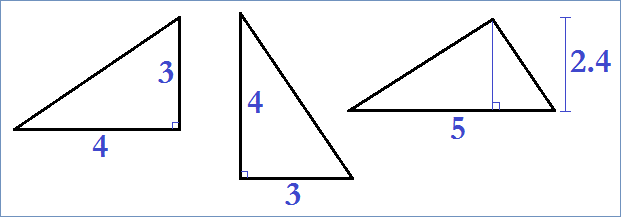# How to Compute the Area of a Triangle: Four Methods

There are many ways to compute the area of a triangle if you know several of its dimensions. You can calculate a triangle's area if you know:

(1) the length of all three sides (SSS)
(2) the length of two sides and the angle between (SAS)
(3) the length of one side and the two adjacent angles (ASA)
(4) the lengths of the base and height.

When you need to figure out the area of a triangle for a construction project or science application, use one of these four methods.

## Side Side Side (SSS), aka Heron's Formula

If the lengths of a triangle are A, B, and C, then the area of the triangle is given by the formula

Area = sqrt[Z(Z-A)(Z-B)(Z-C)],

where Z = (A + B + C)/2. Equivalently, the area can be written as

Area = (0.25)sqrt[2A2B2 + 2A2C2 + 2B2C2 - A4 - B4 - C4].

For example, if a triangle has sides of length 5, 7 and 9, then the area is

(0.25)sqrt[2(25*49 + 25*81 + 49*81) - 625 - 2401 - 6561]
= (0.25)sqrt[2450 + 4050 + 7938 - 625 - 2401 - 6561]
= (0.25)sqrt
= 17.41.

## Side Angle Side (SAS)

If a triangle has two sides with lengths A and B, and the angle between these two sides is X degrees, then the area of the triangle is given by the equation

Area = (0.5)ABsin(X).

You can use a calculator or a trig table to find the sine of an angle. Let's apply the formula to an example. Suppose a triangle has sides of lengths 10 and 12, and the angle between these sides is 75 degrees. Then the triangle's area is

(0.5)(10)(12)(sin(75))
= (60)(0.9659)
= 57.95.

## Angle Side Angle (ASA)

If you know two of the angles inside a triangle and the length of the side between the angles, you can use a third formula to compute the triangular area. If the length of the side is A and the two adjacent angles are X and Y degrees, then the area is

Area = [A2sin(X)sin(Y)]/[2sin(X+Y)].

For example, suppose a triangle has a side length of 16, and the two adjacent angles are 40 degrees and 45 degrees. Then the area is

[162sin(40)sin(45)]/[2sin(85)]
= [256*0.6428*0.7071]/[2*0.9962]
= 116.3581/1.9924
= 58.4.

## Base and Height

If you know the length of the base and the height perpendicular to the base, then you can use the simplest formula for the area of a triangle:

Area = (0.5)BH,

where B is the length of the base, and H is the altitude or height. For example, suppose a triangle has a base of 21 and a height of 8. Then the area is (0.5)(21)(8) = 84. For this equation, the measures of the angles are irrelevant. Nor does it matter how the triangle is oriented, so long as the base and height are measured perpendicular to one another. See image below:These are three different views of the same triangle. No matter how you orient the triangle to measure the base and height, the area will always come out to be 6.